# Capacitor Start Motors: Diagram & Explanation of How a Capacitor is Used to Start a Single Phase Motor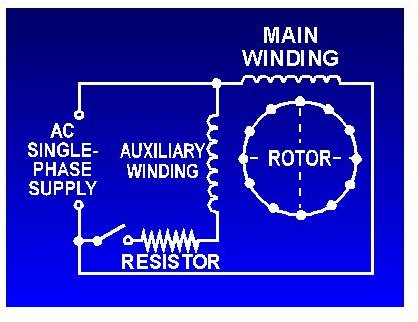The single-phase induction motor can be made to be self-starting in numerous ways. One often-used method is the Split Phase motors. Another method is the Capacitor Start Induction Run Motors.

## Capacitor-Start Induction-Run Motors

We know about the activity of a capacitor in a pure A.C. Circuit. When a capacitor is so introduced, the voltage lags the current by some phase angle. In these motors, the necessary phase difference between the Is and Im is obtained by introducing a capacitor in series with the starter winding. The capacitor used in these motors are of electrolytic type and usually visible as it is mounted outside the motor as a separate unit. (click on an image to enlarge it).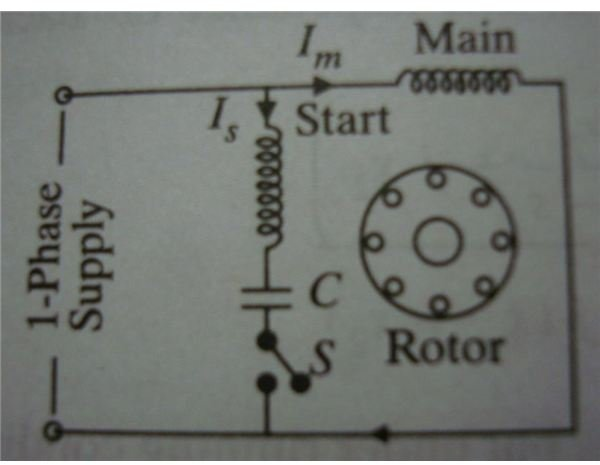During starting, as the capacitor is connected in series with the starter winding, the current through the starter winding Is leads the voltage V, which is applied across the circuit. But the current through the main winding Im, still lags the applied voltage V across the circuit. Thus more the difference between the Is and Im, better the resulting rotating magnetic field.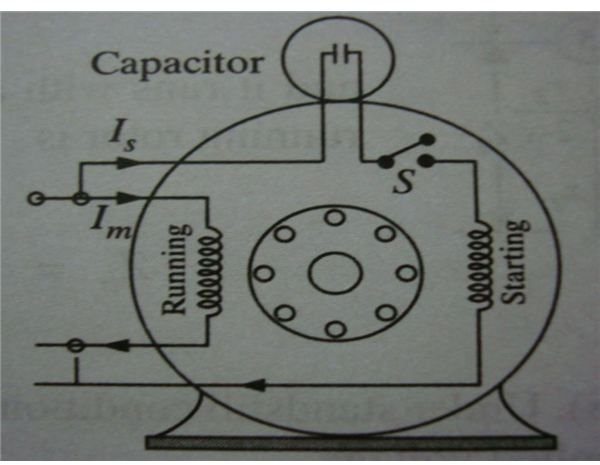When the motor reaches about 75% of the full load speed, the centrifugal switch S opens and thus disconnecting the starter winding and the capacitor from the main winding. It is important to point out from the phasor diagram that the phase difference between Im and Is is almost 80 degrees as against 30 degrees in a split-phase induction motor. Thus a capacitor-start induction-run motor produces a better rotating magnetic field than the split-phase motors. It is evident from the phasor diagram that the current through the starter winding Is leads the voltage V by a small angle and the current through the main winding Im lags the applied voltage. It is to be appreciated that the resultant current I, is small and is almost in phase with the applied voltage V.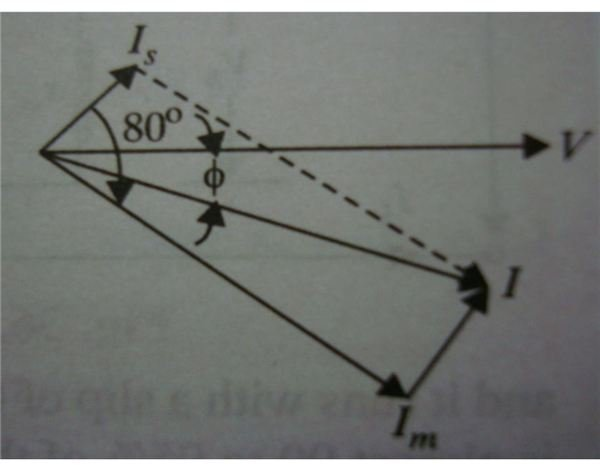The torque developed by a split-phase induction motor is directly proportional to the sine of the angle between Is and Im. Also the angle is 30 degrees in case of split-phase motors. But in the case of capacitor-start induction-run motors, the angle between Is and Im is 80 degrees. It is then obvious that the increase in the angle (from 30 degrees to 80 degrees) alone increases the starting torque to nearly twice the value developed by a standard split-phase induction motor. The speed-torque characteristics curve is exhibiting the starting and running torques of a capacitor-start induction-run motor.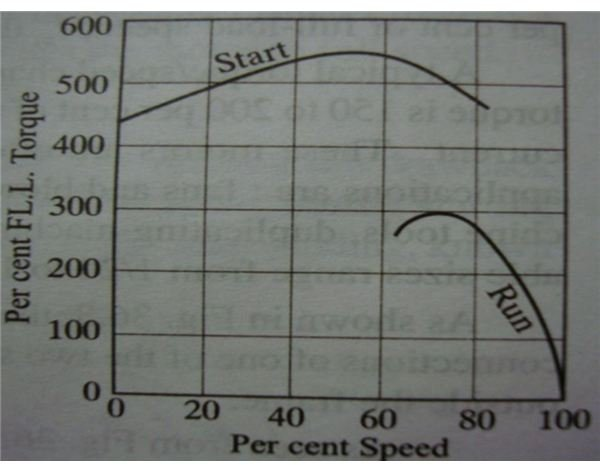## Types of Motors

There are different types of Capacitor-start motors designed and used in various fields. They are as follows:

1. Single-voltage, externally reversible type,
2. Single-voltage, non-reversible type,
3. Single-voltage reversible and with thermostat type,
4. Single-voltage, non-reversible with magnetic switch type,
5. Two-voltage, non-reversible type,
6. Two-voltage, reversible type,
7. Single-voltage, three-lead reversible type,
8. Single-voltage, instantly-reversible type,
9. Two speed type, and
10. Two-speed with two-capacitor type.

These motors can be used for various purposes depending upon the need of the user. The starting, speed/torque characteristics of each of the above motors can be analyzed before employing them in work.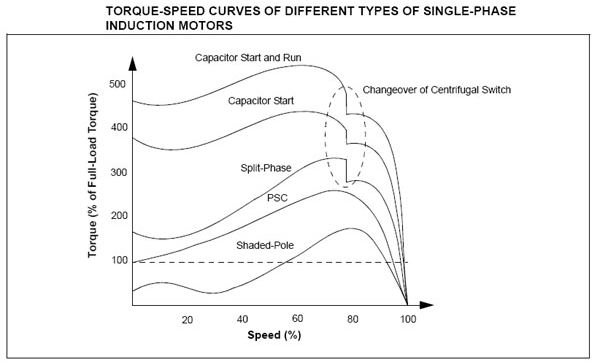My next article is about shaded-pole single-phase motors; you can read it here.

Image Credits:

www.tpub.com

www.allaboutcircuits.com

A/C-D/C Machines by A.K & B.L. Theraja.